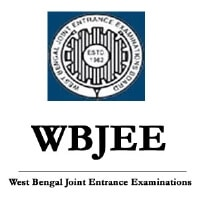Courses

WBJEE Mathematics (Descriptive) - Previous Year Paper, 2011 Notes | Study WBJEE Sample Papers, Section Wise & Full Mock Tests - JEE

JEE: WBJEE Mathematics (Descriptive) - Previous Year Paper, 2011 Notes | Study WBJEE Sample Papers, Section Wise & Full Mock Tests - JEE

The document WBJEE Mathematics (Descriptive) - Previous Year Paper, 2011 Notes | Study WBJEE Sample Papers, Section Wise & Full Mock Tests - JEE is a part of the JEE Course WBJEE Sample Papers, Section Wise & Full Mock Tests.
All you need of JEE at this link: JEE
Page 1

WBJEE - 2011 (Answers & Hints) Mathematics

1. The harmonic mean of two numbers is 4. Their arithemetic mean A and the geometric mean G satisfy the relation 2A + G
2
= 27. find
the numbers.
Ans. (3, 6)
Sol  : Let the number  be a, b
2
A.H G = H4 =
? G
2
= 4A
2A + G
2
= 27 ? 2A + 4A = 27
?
27
A
62
9
==
?  G
2
=  18  ?  G =
18
? a.b = 18
a + b = 9 ?
a6 or a 3
b3b6
==
==
2. If the area of a rectangle is 64 sq. unit, find the minimum value possible for its perimeter.
Ans. 32
Sol. Let the dimesions be a, b
Area = ab
Paimeter = 2(a + b)
We have ab  =  64  ? b =
64
a
Perimeter as function of a
P (a) =
64
2a
a
??
+
??
??
for maxima or minimum
()
2
64
Pa 2 1 0
a
??
' =- =
??
??
? a = ± 8 = 8
()
33
64 2 64
Pa 2 0
a8
×
'' =× = >
P (8) is minimum
Minimum P (8) = 2 ( 8 + 8) = 32
DESCRIPTIVE TYPE QUESTIONS
SUB : MATHEMATICS
Page 2

WBJEE - 2011 (Answers & Hints) Mathematics

1. The harmonic mean of two numbers is 4. Their arithemetic mean A and the geometric mean G satisfy the relation 2A + G
2
= 27. find
the numbers.
Ans. (3, 6)
Sol  : Let the number  be a, b
2
A.H G = H4 =
? G
2
= 4A
2A + G
2
= 27 ? 2A + 4A = 27
?
27
A
62
9
==
?  G
2
=  18  ?  G =
18
? a.b = 18
a + b = 9 ?
a6 or a 3
b3b6
==
==
2. If the area of a rectangle is 64 sq. unit, find the minimum value possible for its perimeter.
Ans. 32
Sol. Let the dimesions be a, b
Area = ab
Paimeter = 2(a + b)
We have ab  =  64  ? b =
64
a
Perimeter as function of a
P (a) =
64
2a
a
??
+
??
??
for maxima or minimum
()
2
64
Pa 2 1 0
a
??
' =- =
??
??
? a = ± 8 = 8
()
33
64 2 64
Pa 2 0
a8
×
'' =× = >
P (8) is minimum
Minimum P (8) = 2 ( 8 + 8) = 32
DESCRIPTIVE TYPE QUESTIONS
SUB : MATHEMATICS
WBJEE - 2011 (Answers & Hints) Mathematics

3. Find the image of the point (–8, 12) with respect to the line 4x + 7y + 13 = 0
Ans. (–16, –2)
Sol.
Image
(h, k)
A
P
(-8, 12)
(h - 8/2, k + 12/2)
(4x + 7y + 13 = 0)
A '
h8 k 12
47 130
22
-+ ?? ? ?
++=
?? ? ?
?? ? ?
2h – 16 +
7k
2
+ 42 + 13 = 0
4h  +  7k  +  78 = 0
4 h 7k 78 .......(i) +=-
2 nd equation, we can get
Slope of  AA' =
7
4
k12 7
n18 4
-
=
+
?  4k – 48 = 75 + 56
4k – 7h = 104 ........(ii)
Solving  (i)  &  (ii)
Equation (i) × 7 + Equation (ii) × 4
?
28h 49k 546
28h 16 k 416
65k 130
+=-
-+ =
=-
k2 =-
h16 =-
A' (– 16, –2) is the image of (–8, 12)
4. How many triangles can be formed by joining 6 points lying on a circle ?
Ans. 20
Sol. Number of triangle

6
C
3
=
6
20
33
=
5. If r
2
= x
2
+ y
2
+ z
2
, then prove that
11 1
yz zx xy
tan tan tan
rx ry rz 2
-- -
?? p ?? ? ?
++ =
?? ?? ? ?
?? ? ?
??
A.
1 13
2
SS
tan
1S
-
?? -
?=
??
-
??Use Code STAYHOME200 and get INR 200 additional OFF Use Coupon Code

Top Courses for JEEWBJEE Sample Papers, Section Wise & Full Mock Tests

4 videos|10 docs|54 tests

Top Courses for JEETrack your progress, build streaks, highlight & save important lessons and more!

,

,

,

,

,

,

,

,

,

,

,

,

,

,

,

,

,

,

,

,

,

,

,

,

,

,

,

;